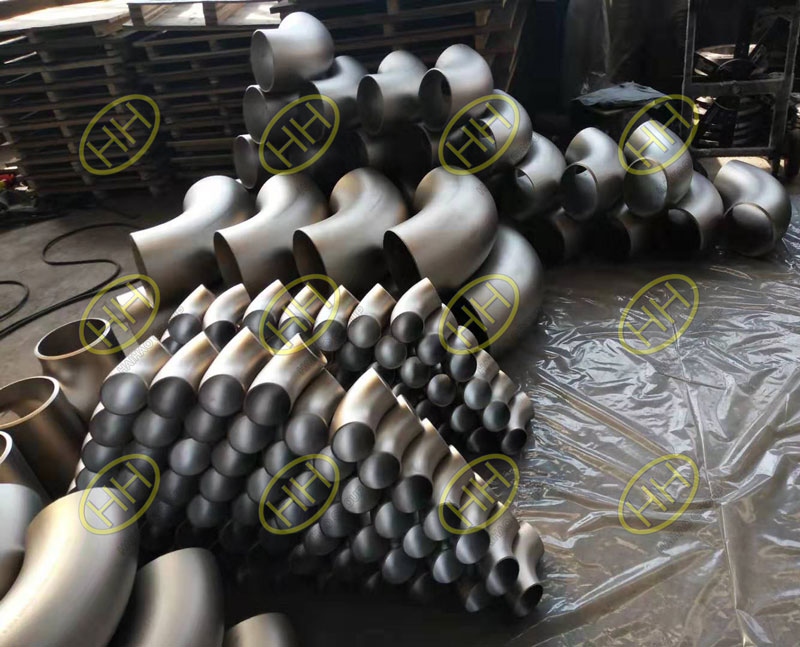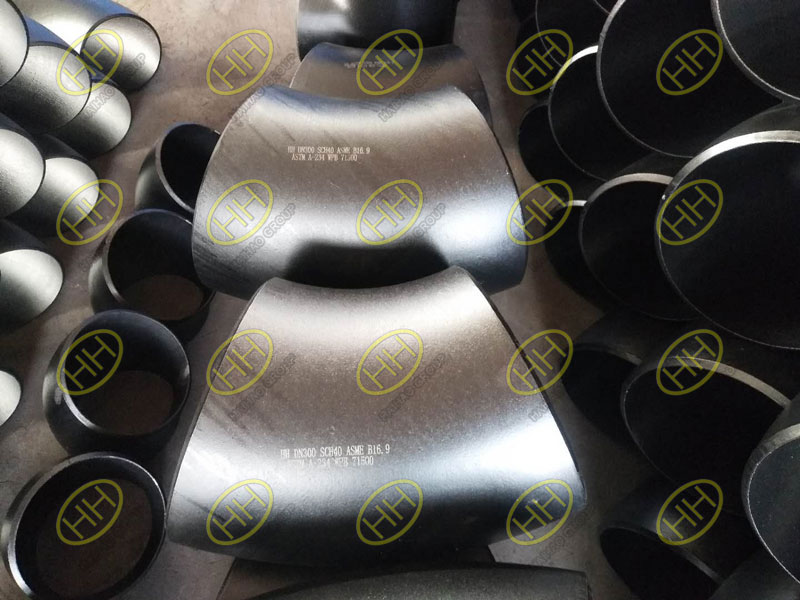Elbows can be divided into long radius elbows and short radius elbows according to the radius of curvature.Long radius elbow refers to the outer diameter of the pipe with a radius of curvature of 1.5 times, namely R = 1.5d.A short radius elbow means that its radius of curvature is equal to the outside diameter of the pipe, namely R = D, where D is the diameter of the elbow and R is the radius of curvature.The normal radius length of the elbow is sometimes used to reduce the resistance loss or wear of the elbow;Use elbows with short radius when installation position is limited.ASME B16.9 ASTM A403 WP304 Long Radius Elbows

From a visual point of view, the short radius elbow is shorter than the long radius elbow and the bend radius of the elbow is very short.Long radius elbow is the most commonly used one, the default is also a long radius, high pressure or high speed it uses a long radius, if it is a solid pipeline requires a large radius elbow.Shorter radii are usually used for low pressure fluids or with limited elbows.If there are no conditions or the preferred long radius elbow is not specified in the contract.

Long radius elbows are the most commonly used bend fittings connected to pipes or pipelines. They are usually called 1.5D elbows. Basically, this type of elbow is selected in the pipeline without special requirements, and it has a wide range of uses. The highest degree of practicality.ASME B16.9 A234 WPB 45 degree short radius elbows

The long radius elbow has a large bending radius (R radius), and the wear during use is smaller than that of the short radius. The strength of the erosion is also reduced a lot, and the resistance is also small (compared with the earlier large multiple elbows and long The difference between radius elbows is similar, because the larger the circle of the elbow, the smaller the influence of all parts, such as the difference between straight pipe and elbow).

Compared with the long radius elbow, the amount of short radius elbow is smaller, and it is mainly used for ship engineering. Although the amount is not large, the position where the short radius elbow is used generally cannot be used for the long radius elbow, so generally Under what circumstances should a short radius elbow be used?

Generally, short-radius elbows are used in conditions where the corners are relatively sharp, and long-radius elbows are used when space is not allowed.

There are many similarities. If they are connected to the pipeline, the function is to change the direction of the pipeline, and the diameter, angle, material, wall thickness, etc. can be consistent.

The difference between the two elbows: as shown in the above two pictures, it can be clearly distinguished that the elbows of the same aperture, wall thickness and material are indeed different. The two elbows are obviously different, one is high and the other is low. Most of the elbows used in normal working conditions are Long-radius elbows, while short-radius elbows can not be used when space is limited, long-radius elbows or sharp turning conditions are used; long-radius elbows are better than short-radius elbows by erosion and wear.

The selection of the two elbows needs to be determined according to the actual working conditions of the pipeline or pipeline, because some positions are both available, and in some working conditions, only one can be selected.

﻿